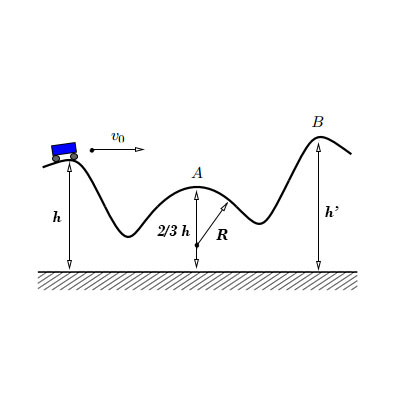# A frictionless roller coaster is given an initial velocity of v_0 at height h = 28 \ m . a) Find...

## Question:

A frictionless roller coaster is given an initial velocity of {eq}v_0 {/eq} at height {eq}h = 28 \ m {/eq}. The radius of curvature of the track at point {eq}A {/eq} is {eq}R = 31 \ m. {/eq}

a) Find the maximum value of {eq}v_0 {/eq} so that the roller coaster stays on the track at {eq}A {/eq} solely because of gravity. The acceleration due to gravity is {eq}9.8 \ m/s^2 {/eq}.

b) Find the value of {eq}h' {/eq} that is necessary if the roller coaster just makes it to point {eq}B {/eq}.## Minimum Velocity to complete the circle:

The minimum velocity fo the object at the top of the circle that is required to complete the circular motion is given by the square root of the product of the acceleration due to gravity and radius of the circle.

(a)

• Minimum velocity at point A {eq}V_{A} = \sqrt{gR} \rm \ m/s \\ V_{A} = \sqrt{9.8 \times 31} \\ V_{A} = 17.43 \rm \ m/s {/eq}

Now, applying the energy conservation between the initial point and point A,

{eq}\begin{align} mgh + \dfrac{1}{2}mv_{o}^{2} &= \dfrac{1}{2}mV_{A}^{2} + mg \left(\dfrac{2h}{3}\right) \\ (9.8 \times 28) + 0.5v_{o}^{2} &= 0.5(17.43)^{2}+ 9.8 \times\dfrac{2 \times 28}{3} \\ v_{o} &= 11 \rm \ m/s \\ \end{align} {/eq}

(b)

Now, applying the energy conseravation between the inital and the final point.

{eq}\begin{align} mgh + \dfrac{1}{2}mv_{o}^{2} &= mgh' \\ (9.8 \times 28) + 0.5(11)^{2} &= 9.8h' \\ h' &= 34.17 \rm \ m \\ \end{align} {/eq}